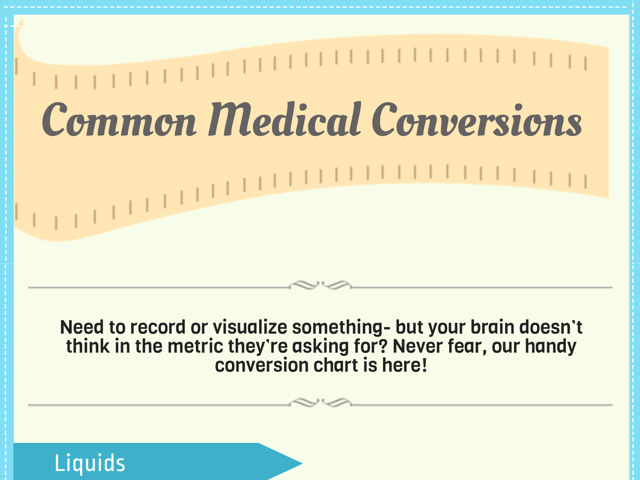# Common Medical Conversions

As a potential nursing student, there is an abundance of information to remember and apply. One area that often proves challenging is conversions of dosages, weights, measures, time, temperatures, and liquid volumes. Given that these are a significant part of the TEAS Exam, understanding them is essential. In this blog post, we’ll discuss some common conversion charts that may prove beneficial.

## Dosage Calculation Conversion Chart

Medication dosages can be represented in several units. This chart can be a quick guide for some common conversions:

Original Unit Equivalent in other units
1 microgram (mcg) 0.001 milligrams (mg)
1 milligram (mg) 1000 micrograms (mcg)
1 milligram (mg) 0.001 grams (g)
1 gram (g) 1000 milligrams (mg)
1 milliliter (ml) 1 cubic centimeter (cc)
1 liter (l) 1000 milliliters (ml)

Please note that dosage calculations require precision and should always be double-checked. In addition to the unit conversions, you should also understand how to calculate dosages based on body weight (mg/kg), body surface area, and other patient-specific factors.

## Weight Conversion Chart

The medical field frequently uses a variety of weight units. Here’s a chart that will help you quickly convert between these units:

Original Unit Equivalent in other units
1 milligram (mg) 0.001 grams (g)
1 gram (g) 1000 milligrams (mg)
1 gram (g) 0.001 kilograms (kg)
1 kilogram (kg) 1000 grams (g)
1 kilogram (kg) 2.20462 pounds (lb)
1 pound (lb) 0.453592 kilograms (kg)

## Liquid Volume Conversion Chart

Liquid measurements are integral to the medical field for activities such as prescribing and administering medication. Use this chart to convert between various units:

Original Unit Equivalent in other units
1 milliliter (ml) 0.001 liters (l)
1 liter (l) 1000 milliliters (ml)
1 liter (l) 1.05669 quarts (qt)
1 quart (qt) 0.946353 liters (l)

Note: 1 milliliter (ml) is approximately equivalent to 1 cubic centimeter (cc)

## Temperature Conversion Chart

Body temperature readings can be in either Fahrenheit or Celsius. Use the following formulas to convert between these units:

Original Unit Conversion Formula
Fahrenheit to Celsius (°F - 32) x 5/9 = °C
Celsius to Fahrenheit (°C x 9/5) + 32 = °F

## Length Measurement Conversion Chart

Length measurements in healthcare are crucial for various purposes, such as calculating body mass index (BMI) or measuring wound size. This chart will assist you in these conversions:

Original Unit Equivalent in other units
1 millimeter (mm) 0.1 centimeters (cm)
1 centimeter (cm) 10 millimeters (mm)
1 centimeter (cm) 0.01 meters (m)
1 meter (m) 100 centimeters (cm)
1 meter (m) 1.09361 yards (yd)
1 yard (yd) 0.9144 meters (m)

## Time Conversion Chart

In healthcare settings and during your TEAS Exam, you may need to convert between different units of time. This chart provides a quick reference:

Original Unit Equivalent in other units
1 minute (min) 60 seconds (s)
1 hour (hr) 60 minutes (min)
1 day 24 hours (hr)
1 week 7 days
1 month (average) ~30.44 days
1 year 12 months

Please note, the month conversion is an average and will vary depending on the specific month (28-31 days). The year is considered a non-leap year (365 days).

## Good Luck On Your TEAS Exam!

Understanding these conversions is crucial for nursing students. Memorizing these charts will not only help you on the TEAS Exam but also during your nursing practice. However, always remember to check the conversion ratios in your local medical settings as they can have minor differences. Happy studying!